Next: 3. Problems with the Up: 2. Identification penalties Previous: 2.2 Linear orbit identification

## 2.3 Restricted orbit identification

For the reasons discussed in Section 3, it is not always possible to use the linear identification theory based upon all 6 orbital elements. The question is how to use the same algorithm on a subset of the orbital elements? The answer is implicit in the arguments presented in [Paper I], Section 2.3, Case 2, which we are going to use without repeating the formal proofs.

Let us suppose that the vector of estimated parameters is split into two components, along linear subspaces of the parameter space: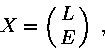whereare elements of interest. The normal and covariance matricesandare decomposed as follows:Then the uncertainty offor arbitrarycan be described by the penalty, with respect to the minimum point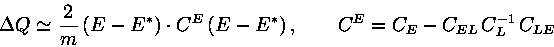and by the marginal covariance matrix.

Note that the marginal normal matrix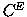is not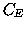and that to obtain the penalty of the above formula as a function of, the value ofhas to be changed with respect to the nominal solutionof the unrestricted problem, by an amount which is a function of:Let us apply this restricted penalty formula to the restricted identification problem. Let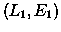and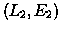be the nominal solutions for the two arcs considered separately, andand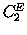the corresponding marginal normal matrices. The variablesare given as function ofby:(3)

By the same formalism of the previous subsection:with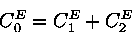Note thatis not the same as the complete minimum penalty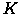of the previous section. The estimateof the minimum penalty is obtained by assuming that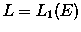in the computation ofwhile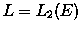in the computation of. Thus there is, in general, no complete solution with a single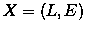to be fit to the observations of both arcs with penalty, but such value is obtained by using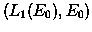in the first arc,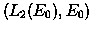in the second arc,being the proposed restricted identification.

We claim that:is the minimum of the penalty over the space of variables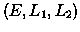, whileis the minimum of the same penalty over the same space but with the additional constraint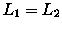, and the minimum of a function can only increase when constraints are added.

In conclusion, the proposed restricted identificationis not a complete identification, and the corresponding minimum penaltyis not the full penalty to be paid to achieve a full identification. This procedure is, however, a good way to filter the possible identifications because: if a couple can be discarded as a possible identification with the restricted computation, becauseis too large, then it does not need to be tested with the complete algorithm.

Note that it is also possible to define a constrained identification algorithm, based upon the conditional covariance matrices and the algorithm for constrained optimisation on linear subspaces, as outlined in [Paper I], Section 2.3.Next: 3. Problems with the Up: 2. Identification penalties Previous: 2.2 Linear orbit identification

Maria Eugenia Sansaturio
1999-05-20